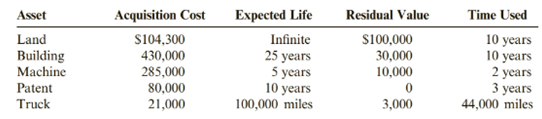Chapter 7, Problem 63E### Cornerstones of Financial Accounti...

4th Edition
Jay Rich + 1 other
ISBN: 9781337690881

#### Solutions

Chapter
Section### Cornerstones of Financial Accounti...

4th Edition
Jay Rich + 1 other
ISBN: 9781337690881
Textbook Problem
13 views

# Balance Sheet PresentationThe following information relates to the assets of Westfield Semiconductors as of December 31, 2019. Westfield uses the straight-line method for depreciation and amortization.Required:Use the information above to prepare the property, plant, and equipment and intangible assets portions of a classified balance sheet for Westfield.

To determine

Concept introduction:

Straight-line Depreciation:

Depreciation is done by allocating the cost of the fixed assets other than land to expense over the useful life of the asset. The most commonly used method of depreciation is straight line method. In this method every year until the useful life of the asset, equal amount of assets cost to depreciation expense is allocated. The formula to calculate straight line depreciation is:

Straight line depreciation = (cost − residual value) / expected useful life

Balance Sheet:

It is a statement of the financial position of the company which specifies the assets, liabilities and equity at a specified date.

Prepare property, plant and equipment and intangible assets portions balance sheet for Westfield.

Explanation

To prepare the property, plant and equipment and intangible assets portions of a classified balance sheet for Westfield first we have to determine the book value of each assets. Now let us calculate the accumulated depreciation of each asset to determine the book value:

Depreciation for land    = (cost − residual value) / useful life   = (104300 − 100000) / 10 = 430
Book value of land    = Cost − Accumulated depreciation   = 104300 − 430 = 103870
Depreciation for Building    = (cost − residual value) / useful life   = (430000 − 30000) / 10 = 40000
Book value of Building    = Cost − Accumulated depreciation   = 430000 − 40000 = 390000
Depreciation for Machine    = (cost − residual value) / useful life   = (285000 − 10000) / 2 = 137500
Book value o...

### Still sussing out bartleby?

Check out a sample textbook solution.

See a sample solution

#### The Solution to Your Study Problems

Bartleby provides explanations to thousands of textbook problems written by our experts, many with advanced degrees!

Get Started

#### Find more solutions based on key concepts